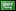### 2 simple labs in Matlab

• Posted at : 1 month ago
• Post Similar Project
2 /Hr

Estimated Hours - 3
Budget
15
Proposals
162
Views
Expired
Status
Skills Required

Posted By -

AASaudi Arabia
0.0
Projects Posted : 2
Projects Paid : 0
Services Purchased : 0
Total Spent :
0
Feedbacks : 0 %

#### Project Details hide (-)

Lab 2
Modify the bisection method code that presented in the

LAB to include Maximum Number of iteration (No), and
check for the root that found is it true root or not
Run your code for the function
F(x)=3*x^6+15*x^5-10*x3+4*x

function p bisection (f,a,b )
if f(a)*f (b) >0
disp ('Wrong choice bro')
else p = (a + b)/2;
err = abs (f (p) );
while err > le-7
if f(a)*f(p) <0
b = p;
else a = p;
end p = (a + b) /2;
err = abs (f (p));
end
end

LAB #3
Run the bisection method code and Fixed-point iteration
method for the function
F(x)=3*x^6+15*x^5-10*x3+4*x, for tol=0.01%
Then find
1.
Number iteration required to stop
2.
Determine the accuracy of the calculated root
3. Running time required by each method